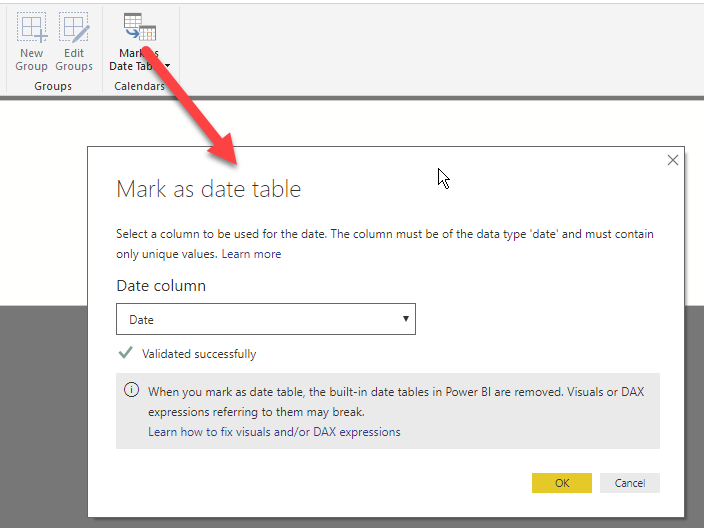A date table is required for most time intelligence calculations such as year-to-date, previous year or moving averages. If a data model does not already have a date table, it is possible to create one using a calculated table and some basic DAX code.

The date table needs to follow a few rules:

• All the dates – from the first to the last day of each year – need to be present.
• Each date gets its own row.
• There are no holes allowed, even if a date is not referenced by an event.
• The table needs to include one DateTime type column.

There are a couple of functions in DAX that create a simple date table: CALENDAR and CALENDARAUTO. Both functions return a table with a single column named “Date” and a list of values for the dates. CALENDAR requires the boundaries of the set of dates, whereas CALENDARAUTO searches among all the dates in the data model and automatically finds the first and last year referenced within the model.

Unfortunately, none of these two functions is perfect. In fact, CALENDARAUTO searches in all the date columns of the data model, including – for example – customer birth dates. As a result, CALENDARAUTO might create a date table containing many irrelevant years. On the other hand, CALENDAR requires the computing of the MIN and MAX date of all transactions available (e.g. sales) and then moves them respectively to the first and last day of these given years.

Therefore, the easiest way of creating a simple calendar table is to rely on CALENDARAUTO to find all dates available, and then remove from all the dates found the ones that don’t fall inside the period of interest. For example, the following expression is a good starting point for a date table:

```Date =
VAR MinYear = YEAR ( MIN ( Sales[Order Date] ) )
VAR MaxYear = YEAR ( MAX ( Sales[Order Date] ) )
RETURN
FILTER (
CALENDARAUTO( ),
AND ( YEAR ( [Date] ) >= MinYear, YEAR ( [Date] ) <= MaxYear )
),
"Calendar Year", "CY " & YEAR ( [Date] ),
"Month Name", FORMAT ( [Date], "mmmm" ),
"Month Number", MONTH ( [Date] )
)
```

By using the two variables MinYear and MaxYear, the remaining part of the code does not depend on the data model. Thus, this code can easily be pasted into another data model and adapted to various needs with minor changes.

Once the date table is in place, it is a good idea to mark it as a date table as shown here:This both simplifies the code to author time intelligence calculations and automatically disables the auto Date/Time feature.

The code snippet shown above is just a starting point for a real date table. Specific requirements will help achieve a more complete definition. If, for example, the day of the week is required, it is possible to add columns with the weekday as a string or as a number. The same applies to the definition of quarter, fiscal months, years, and any other column required.

```Date =
VAR MinYear = YEAR ( MIN ( Sales[Order Date] ) )
VAR MaxYear = YEAR ( MAX ( Sales[Order Date] ) )
RETURN
FILTER (
CALENDARAUTO( ),
AND ( YEAR ( [Date] ) >= MinYear, YEAR ( [Date] ) <= MaxYear )
),
"Calendar Year", "CY " & YEAR ( [Date] ),
"Month Name", FORMAT ( [Date], "mmmm" ),
"Month Number", MONTH ( [Date] ),
"Weekday", FORMAT ( [Date], "dddd" ),
"Weekday number", WEEKDAY( [Date] ),
"Quarter", "Q" & TRUNC ( ( MONTH ( [Date] ) - 1 ) / 3 ) + 1
)
```

A more complete example of a date table is available in the article Reference Date Table in DAX and Power BI.

CALENDARReturns a table with one column of all dates between StartDate and EndDate.

`CALENDAR ( <StartDate>, <EndDate> )`

CALENDARAUTOReturns a table with one column of dates calculated from the model automatically.

`CALENDARAUTO ( [<FiscalYearEndMonth>] )`

MINReturns the smallest value in a column, or the smaller value between two scalar expressions. Ignores logical values. Strings are compared according to alphabetical order.

`MIN ( <ColumnNameOrScalar1> [, <Scalar2>] )`

MAXReturns the largest value in a column, or the larger value between two scalar expressions. Ignores logical values. Strings are compared according to alphabetical order.

`MAX ( <ColumnNameOrScalar1> [, <Scalar2>] )`